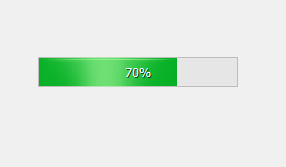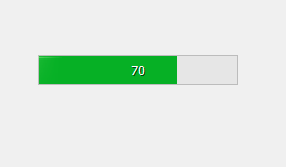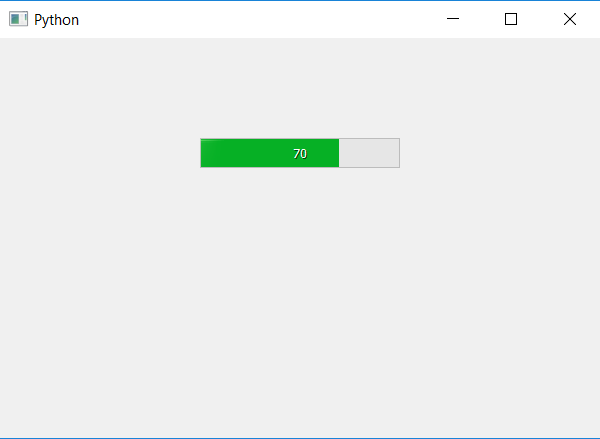# PyQt5 – How to remove the percentage symbol from the Progress Bar ?

• Last Updated : 22 Apr, 2020

In this article we will see how to remove the percentage symbol the progress bar i..e ‘%’, when we create progress bar by default percentage and percentage symbol appear.

In order to remove only the percentage symbol we have to change the format of the progress bar, below is how normal progress bar looks like vs after removing the percentage symbol looks like.Attention geek! Strengthen your foundations with the Python Programming Foundation Course and learn the basics.

To begin with, your interview preparations Enhance your Data Structures concepts with the Python DS Course. And to begin with your Machine Learning Journey, join the Machine Learning - Basic Level Course

By default the formatting is ‘%p%’ here p represents the percentage, if we change it to ‘%p’ it will show only number not the percentage symbol.

Below is the implementation.

 `# importing libraries``from` `PyQt5.QtWidgets ``import` `*` `from` `PyQt5 ``import` `QtCore, QtGui``from` `PyQt5.QtGui ``import` `*` `from` `PyQt5.QtCore ``import` `*` `import` `sys`` ` ` ` `class` `Window(QMainWindow):`` ` `    ``def` `__init__(``self``):``        ``super``().__init__()`` ` `        ``# setting title``        ``self``.setWindowTitle(``"Python "``)`` ` `        ``# setting geometry``        ``self``.setGeometry(``100``, ``100``, ``600``, ``400``)`` ` `        ``# calling method``        ``self``.UiComponents()`` ` `        ``# showing all the widgets``        ``self``.show()`` ` `    ``# method for widgets``    ``def` `UiComponents(``self``):``        ``# creating progress bar``        ``bar ``=` `QProgressBar(``self``)`` ` `        ``# setting geometry to progress bar``        ``bar.setGeometry(``200``, ``100``, ``200``, ``30``)`` ` `        ``# setting the value``        ``bar.setValue(``70``)`` ` `        ``# setting alignment to center``        ``bar.setAlignment(Qt.AlignCenter)`` ` `        ``# removing percentage symbol``        ``bar.setFormat(``"% p"``)`` ` `App ``=` `QApplication(sys.argv)`` ` `# create the instance of our Window``window ``=` `Window()`` ` `# start the app``sys.exit(App.``exec``())`

Output :My Personal Notes arrow_drop_up